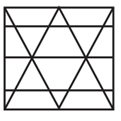# SSC Reasoning Ability Practice Questions (Day-3)

Dear Aspirants, Here we have given the Important SSC CGL Exams 2018 Practice Test Papers. Candidates, those who are preparing for SSC CGL Exams 2018 can practice these questions to get more confidence to Crack SSC CGL Exams 2018 Examination.

[WpProQuiz 4031]

1) Aarush’s birthday is on Thursday 27th April. On what day of the week will be Mitul’s birthday in the same year, if Mitul was born on 28th September?

a) Monday

b) Thursday

c) Wednesday

d) Tuesday

2) The weights of 4 boxes are 20 kg, 30 kg, 40 kg and 60 kg. Which of the following cannot be the total weight, in kilogram, of any combination of these boxes and in a combination a box can be used only once?

a) 150

b) 110

c) 130

d) 140

3) From the given words, select the word which cannot be formed using the letters of the given word.

HUMBLING

a) BLUE

b) NUMB

c) LUNG

d) GLUM

4) If FOREIGN is coded as ULIVRTM, then how will SAP be coded as.

a) NOL

b) PLQ

c) GYP

d) HZK

5) In a certain code language, ‘+’ represents ‘×’,  ‘–‘ represents ‘+’, ‘×’ represents ‘÷’ and ‘÷’ represents ‘–’, What is the answer to the following question?

25 + 10 ÷240×16 = ?

a) 85

b) 235

c) 12

d) 20

Direction  (Q. No 6-8) In the following questions, select the odd word/ letters/ number from the given alternatives.

6)

a) Football

b) Hockey

c) Carrom

d) Cricket

7)

a) ACE

b) FHJ

c) QTW

d) KMO

8)

a) 121

b) 44

c) 66

d) 111

9) Two motorcyclists P and Q start from the same point. P rides 11 km West, then turns South and rides 16 km, then turns to his right and rides 14 km. Q rides 30 km South, then turns to his right and rides 25 km. Where is Q with respect to P now?

a) 14 km North

b) 14 km South

c) 44 km South

d) 4 km North

10) In the question, a statement is given, followed by two Arguments, I and II. You have to consider the statement to be true even if it seems to be at variance from commonly known facts. You have to decide which of the given arguments, if any, is a strong argument.

Statement:

Should mango export be banned to bring down domestic prices?

Arguments:

I. Yes, environmentalists and dieticians to encourage eating only local fruits.

II. No, exports bring in valuable foreign currency.

a) If only Argument I is strong

b) If only Argument II is strong

c) If both Arguments I and II are strong

d) If neither Argument I nor II is strong

11) Three positions of a cube are shown below. What will come opposite to face containing ‘T’?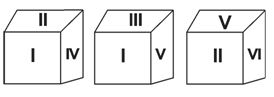a) VI

b) IV

c) II

d) V

12) In the given figure, how many pens are blue?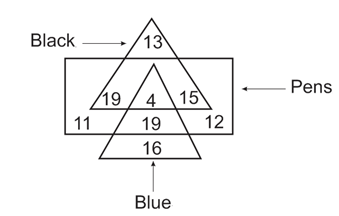a) 23

b) 20

c) 19

d) 25

13) Which answer figure will complete the pattern in the question figure?

Question Figure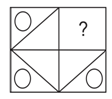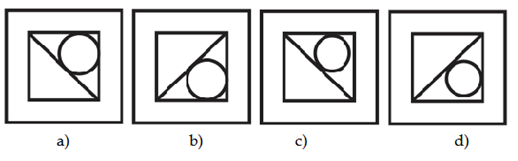14) Which answer figure will complete the pattern in the question figure?

Question Figure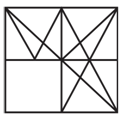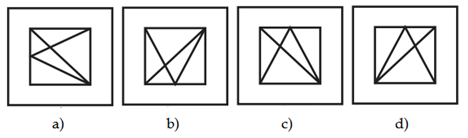15) From the given answer figures, select the one in which the question figure is hidden/ embedded.

Question Figure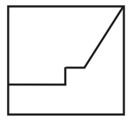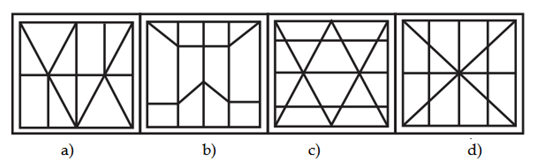The day of Aarush’s birthday (27th April) = Thursday

The number of days from 28th April to 28th September

= 3 + 31 + 30 + 31 + 31 + 28 = 154

Odd days = 154/7

Remainder = 0

The day of Mitul’s birthday

= Thursday + 0 = Thursday

Weight of four boxes = 20 kg, 30 kg, 40 kg and 60 kg

Total weight of four boxes

= 20 + 30 + 40 + 60 = 150 kg

Possible combination,

150 – 20 = 130 kg

150 – 30 = 120 kg

150 – 40 = 110 kg

150 – 60 = 90 kg

According to the options, combination of 140 kg cannot be formed.

The word BLUE cannot be formed using the letters of the given word because the word HUMBLING does not have letter E.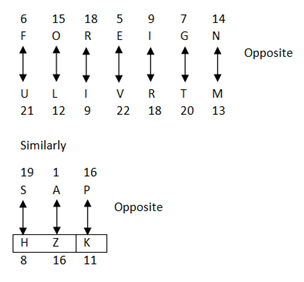According to the question,

? = 25 +10 ÷240 ×16

On changing the signs,

? = 25 × 10 – 240 ÷ 16

On using BODMAS,

? = 25 × 10 – 15

? = 250 – 15 = 235

Direction (6-8) :

Rest of options includes 11 players and outdoor games.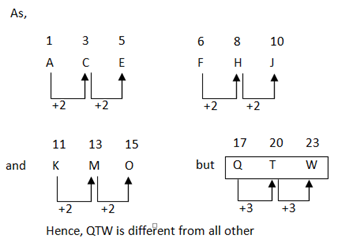Rest of options is multiple of 11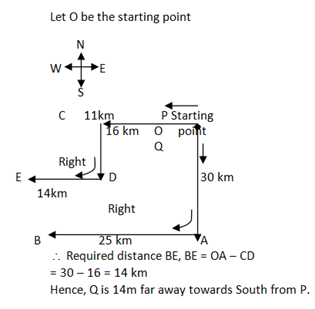Exportation of mangoes should not be banned because such exports bring a valuable foreign currency. Hence, Argument II is strong.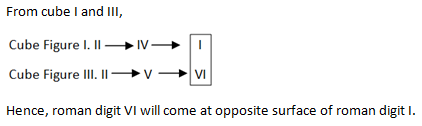Number of blue pens = 4 + 19 = 23

Hence, there are 23 pens according to the figure.

Answer figure c) will complete the question figure.

Answer figure: d) will complete the question figure.